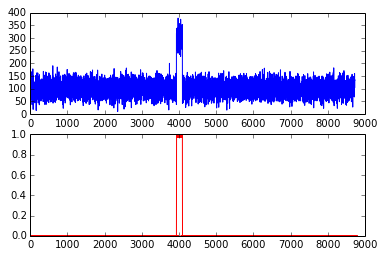## 用Python做单变量数据集的异常点分析

### 2014年6月12日 18:03

```import numpy as np
import pandas as pd

Pandas 是一个常用的数据分析的Python库，提供对数据的加载，清洗，抽取，变形等操作。Pandas依赖numpy，numpy提供了基于列/多维数组（List/N-D Array）的数据结构的操作。许多科学计算和数据分析的库都依赖于numpy。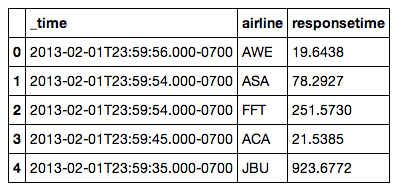```array(['AAL', 'ACA', 'AMX', 'ASA', 'AWE', 'BAW', 'DAL', 'EGF', 'FFT',
'JAL', 'JBU', 'JZA', 'KLM', 'NKS', 'SWA', 'SWR', 'TRS', 'UAL', 'VRD'],
dtype='|S3')```

`dd = df.query('airline=="KLM"') ## 得到法航的数据`

`dd.responsetime.describe()`

```count    1724.000000
mean     1500.613766
std       100.085320
min      1209.766800
25%      1434.084625
50%      1499.135000
75%      1567.831025
max      1818.774100
Name: responsetime, dtype: float64```

### 基于标准差得异常检测

• 95.449974面积在平均数左右两个标准差的范围内

• 99.730020%的面积在平均数左右三个标准差的范围内

• 99.993666的面积在平均数左右三个标准差的范围内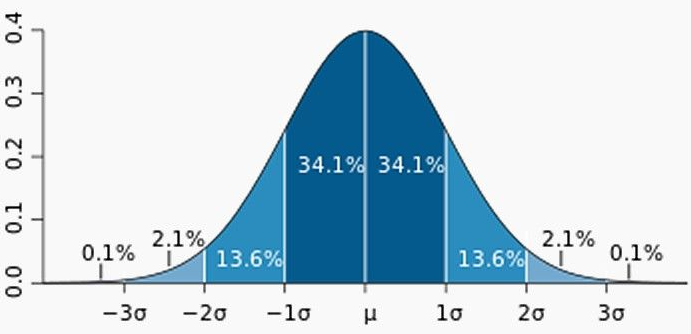```def a1(dataframe, threshold=.95):
d = dataframe['responsetime']
dataframe['isAnomaly'] = d > d.quantile(threshold)
return dataframe
print a1(dd)```

```                             _time airline  responsetime isAnomaly
20    2013-02-01T23:57:59.000-0700     KLM     1481.4945     False
76    2013-02-01T23:52:34.000-0700     KLM     1400.9050     False
124   2013-02-01T23:47:10.000-0700     KLM     1501.4313     False
203   2013-02-01T23:39:08.000-0700     KLM     1278.9509     False
281   2013-02-01T23:32:27.000-0700     KLM     1386.4157     False
336   2013-02-01T23:26:09.000-0700     KLM     1629.9589     False
364   2013-02-01T23:23:52.000-0700     KLM     1482.5900     False
448   2013-02-01T23:16:08.000-0700     KLM     1553.4988     False
511   2013-02-01T23:10:39.000-0700     KLM     1555.1894     False
516   2013-02-01T23:10:08.000-0700     KLM     1720.7862      True
553   2013-02-01T23:06:29.000-0700     KLM     1306.6489     False
593   2013-02-01T23:03:03.000-0700     KLM     1481.7081     False
609   2013-02-01T23:01:29.000-0700     KLM     1521.0253     False
666   2013-02-01T22:56:04.000-0700     KLM     1675.2222      True
...   ...   ...   ...```

1. 方法a1定义了一个异常检测的函数

2. dataframe['responsetime']等价于dataframe.responsetime,该操作取出responsetime这一列的值

3. d.quantile(threshold)用正态分布假定返回位于95%的点的值，大于该值得点都落在正态分布95%之外

4. d > d.quantile(threshold)是一个数组操作，返回的新数组是responsetime和threshold的比较结果，[False,False,True,... ... False]

5. 然后通过dataframe的赋值操作增加一个新的列，标记所有的异常点。

```import matplotlib.pyplot as plt
da = a1(dd)
fig = plt.figure()
ax1.plot(da['responsetime'])
ax2.plot(da['isAnomaly'])```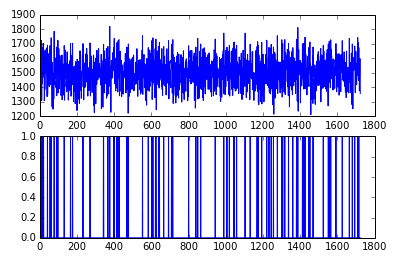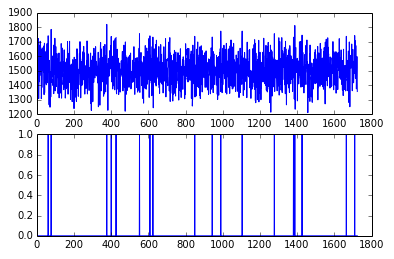### 基于ZSCORE的异常检测

zscore的计算如下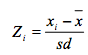sd是标准差，X是均值。一般建议门限值取为3.5

```def a2(dataframe, threshold=3.5):
d = dataframe['responsetime']
zscore = (d - d.mean())/d.std()
dataframe['isAnomaly'] = zscore.abs() > threshold
return dataframe```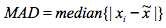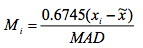```def a3(dataframe, threshold=3.5):
dd = dataframe['responsetime']
zscore = ((dd - dd.median())* 0.6475 /MAD).abs()
dataframe['isAnomaly'] = zscore > threshold
return dataframe```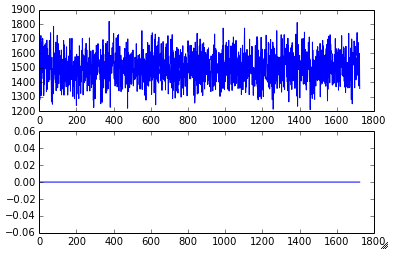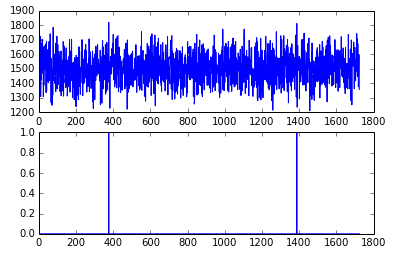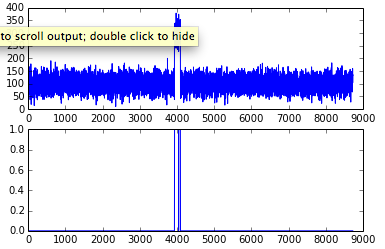### 基于KMEAN聚集的异常检测

```preresponse = 0
newcol = []
newcol.append(0)
for index, row in dd.iterrows():
if preresponse != 0:
newcol.append(preresponse)
preresponse = row.responsetime
dd["t0"] = newcol
plt.scatter(dd.t0,dd.responsetime)```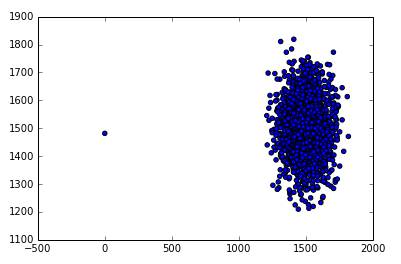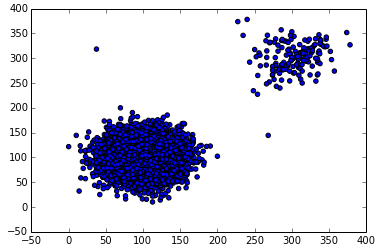```def a4(dataframe, threshold = .9):
## add one dimention of previous response
preresponse = 0
newcol = []
newcol.append(0)
for index, row in dataframe.iterrows():
if preresponse != 0:
newcol.append(preresponse)
preresponse = row.responsetime
dataframe["t0"] = newcol
## remove first row as there is no previous event for time
clf = cluster.KMeans(n_clusters=2)
X=np.array(dd[['responsetime','t0']])
cls = clf.fit_predict(X)
freq = itemfreq(cls)
(A,B) = (freq[0,1],freq[1,1])
t = abs(A-B)/max(A,B)
if t > threshold :
## "Anomaly Detected!"
index = freq[0,0]
if A > B :
index = freq[1,0]
dd['isAnomaly'] = (cls == index)
else :
## "No Anomaly Point"
dd['isAnomaly'] = False
return dd```

```clf = cluster.KMeans(n_clusters=2)
X=np.array(dd[['responsetime','t0']])
cls = clf.fit_predict(X)```

cluster.KMeans返回一个预测模型，我们假定有两个聚集。你可以试着加大聚集的数量，结果没什么影响。

dd[['responsetime','t0']]返回一个2*n的数组，并赋值给X，用于聚集计算。

fit_pridict方法是对X做聚集运算,并计算每一个点对应的聚集编号。

`freq = itemfreq(cls)`

itemfreq返回聚集结果中每一个聚集的发生频率，如果其中一个比另一个显著地多，我们则认为那个少得是异常点聚集。